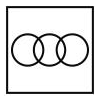0
8515

# Venn Diagram Questions for SSC CGL PDF

Download SSC CGL Venn Diagram Questions & answers PDF. Top 10 SSC CGL questions based on asked questions in previous exam papers very important for the SSC exam.

Question 1: Identify the diagram that best represents the relationship among the given classes.
Animal, Leopard, Lion

a)

b)

c)

d)

Question 2: Identify the diagram that best represents the relationship among the given classes.
Country, State, City

a)

b)

c)

d)

Question 3: Identify the diagram that best represents the relationship among the given classes.
Professionals, Chartered Accountant, Female

a)

b)

c)

d)

Question 4: Identify the diagram that best represents the relationship among the given classes.
Pet animals, Dog, Cat

a)

b)

c)

d)

Question 5: Identify the diagram that best represents the relationship among classes given below
Tennis fans, Cricket players, Students

a)

b)

c)

d)

Question 6: Identify the diagram that best represents the relationship among classes given below
Men, Rodents and living beings

a)

b)

c)

d)

Question 7: Choose the diagram which most correctly captures the relation between the following:
Female, Mother, Doctor, Teacher

a)

b)

c)

d)

Question 8: Identify the diagram that best represents the relationship among classes given below
Males, Fathers, Engineers

a)

b)

c)

d)

Question 9: Identify the diagram that best represents the relationship among classes given below
Minutes, Days, Months

a)

b)

c)

d)

Question 10: Identify the diagram that best represents the relationship among the given classes.
Cricketer, Male, Actor

a)

b)

c)

d)

Both leopard and lion are animals but are completely different from each other.

Thus, the third figure best describes above relationship.

=> Ans – (C)

All cities are part of a state and all states are part of a country.

Thus, the last figure perfectly describes the above relationship.

=> Ans – (D)

Both professionals and Chartered Accountants can be female, and all chartered accountants are professionals, hence the second diagram best describes above relationship.

=> Ans – (B)

All dogs are pet animals, similarly all cats are pet animals, but dogs are different from cats.

Thus, the first figure best represents above relationship.

=> Ans – (A)

A student can be both a tennis fan and a cricket player. Similarly, a tennis fan can be both a student and a cricket player.

Thus, all of the three are interrelated and first option best describes above relationship.

=> Ans – (A)

Rodents and men both come under the category of living beings but are completely different from each other. Thus, the third figure best describes above relationship.

=> Ans – (C)

Option A correctly captures the relationship between the given things. Mother should be a subset of Female. The other sets will have overlapping regions and would not have a subset-superset relationship.

All fathers are definitely males, and the engineers can be either males or fathers.

Thus, the third figure best represents the above relationship.

=> Ans – (C)

Days are formed by minutes and months by days, thus the first figure best describes above relationship.

=> Ans – (A)